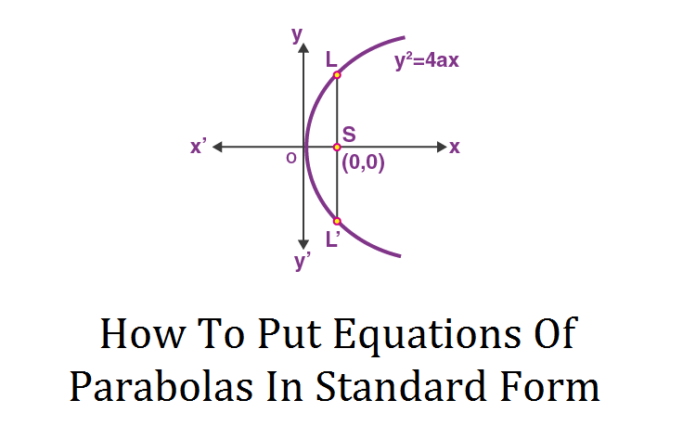# How To Put Equations Of Parabolas In Standard Form?

0
180In mathematics, a parabola is something that looks like an arc of a circle. But do you have any idea why we use parabolas? Let me tell you. Parabolas have very vast domains in the field of telecommunications, architecting, and others. This makes them very crucial to understand so that you may get a firm grip on the various phenomena in which there is a proper use of parabolas. But the very first and important thing here is that you must have a sound knowledge of writing the equation of the parabola in the standard form.

An online standard form calculator by calculator-online.net helps you in determining the equation of the parabola in its standard form. This free standard notation calculator provides you an edge of conversion among various numbers in scientific, real, and engineering notations so that you may not feel any hurdle when writing parabola equations.

In this article, I will be discussing how to write a parabola equation in its standard form. Give a read!

## The Standard Form Of Parabola Equation:

The second-degree equation actually has a resemblance with the standard form that is used to represent the parabola. The equation is as follows:

$$y = ax^{2} + bx + c$$

From this equation, we have two key points:

1) Look at the first number a. What do you think it represents? Do not worry if you did not get it. Let me tell you in actuality, this number decides the shape of the parabola. If it is a negative number, the parabola will open downwards. If it is a positive number, the parabola will open upwards.

Mathematically:

$$a > 0 \hspace{0.25in} \text{Opens Upwards}$$

$$a < 0 \hspace{0.25in} \text{Opens Downwards}$$

The standard notation calculator assists you in finding whether this number is positive or negative in a glimpse of an eye.

2) The second thing here is that if you use both the numbers a and b, they will provide you with the axis of symmetry. The axis of symmetry is the line that acts as a mirror for a parabola. It lies outside of the parabola. The parabola drawn on either side of this lie is exactly the image of the other side. The mathematical notation that you can use to find the axis of symmetry from the standard form of the parabola equation is as follows:

$$x = -\frac{b}{2a}$$

### Example:

Write down the standard form of the following parabola equation:

$$y = left(x-1\right)^2 + 1$$

Solution:

Here we have:

$$y = left(x-1\right)^2 + 1$$

$$y = x^{2} + 1 – 2x + 1$$

$$y = x^{2} – 2x + 2$$

Which is the required standard form. How simple is that! With the help of a free standard form calculator, you will be able to write this form more fastly and accurately.

## Wrapping It Up:

Parabolas have many vast applications. They are widely used to construct parabolic antennas, ballistic missiles, and even microphones that reflect the signal for proper communications. This makes them very crucial terms to have a sound knowledge of. Also, the use of the standard form calculator has also been enlightened so that you people may not feel difficulty in determining the numbers to write the equation of the parabola in standard form.